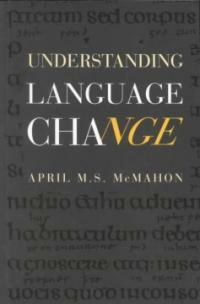> Detail View

# Detail View## Understanding language change (Loan 11 times)

Material type
단행본
Personal Author
McMahon, April M. S.
Title Statement
Understanding language change / April M.S. McMahon.
Publication, Distribution, etc
Cambridge ;   New York :   Cambridge University Press ,   1994.
Physical Medium
xi, 361 p. ; 24 cm.
ISBN
0521446651 (pbk.) 0521441196 (hc)
Bibliography, Etc. Note
Includes bibliographical references (p. 341-352) and index.
Linguistic change.
 000 00702pamuuu200229 a 4500 001 000000019519 005 19950127114350.0 008 930419s1994 enk b 001 0 eng 010 ▼a 93008121 020 ▼a 0521446651 (pbk.) 020 ▼a 0521441196 (hc) 035 ▼a 93008121 040 ▼a DLC ▼c DLC ▼d DLC 050 0 0 ▼a P142 ▼b .M38 1994 082 0 0 ▼a 417/.7 ▼2 20 090 ▼a 417.7 ▼b M263u 100 1 0 ▼a McMahon, April M. S. 245 1 0 ▼a Understanding language change / ▼c April M.S. McMahon. 260 0 ▼a Cambridge ; ▼a New York : ▼b Cambridge University Press , ▼c 1994. 300 ▼a xi, 361 p. ; ▼c 24 cm. 504 ▼a Includes bibliographical references (p. 341-352) and index. 650 0 ▼a Linguistic change.

### Holdings Information

No. Location Call Number Accession No. Availability Due Date Make a Reservation Service
No. 1 Location Call Number 417.7 M263u Accession No. 111012109 Availability Available Due Date Make a Reservation Service
No. 2 Location Call Number 417.7 M263u Accession No. 111024857 Availability Available Due Date Make a Reservation Service

### Contents information

```
CONTENTS
Preface and acknowledgements = xi
1 Introduction = 1
1.1 Linguistic similarities and relationships = 1
1.2 Language change and linguistic reconstruction = 6
1.3 Synchrony and diachrony = 9
1.4 The organisation of this book = 11
2 Three views of sound change = 14
2.1 Introduction: types of sound change = 14
2.2 The Neogrammarians = 17
2.3 The Structuralists = 24
2.4 The Generativists = 32
2.5 The question of explanation = 44
3 Sound change 2: the implementation problem = 47
3.1 Introduction = 47
3.2 Lexical diffusion = 47
3.3 Lexical Phonology and sound change = 56
4 Morphological change = 69
4.1 Introduction = 69
4.2 Analogy= 70
4.3 Natural Morphology = 97
5 Syntactic change 1: the Transparency Principle = 107
5.1 Introduction = 107
5.2 Earlier work on syntactic change = 107
5.3 Lightfoot's (1979a) theory of syntactic change = 116
5.4 Reactions and criticisms = 123
5.5 Lightfoot and the principles and parameters model =129
6 Word order change and grammaticalisation: language change and general laws = 138
6.1 Introduction = 138
6.2 Typology and consistency in word order = 139
6.3 Grammaticalisation = 160
7 Semantic and lexical change = 174
7.1 Introduction = 174
7.2 Semantic change = 174
7.3 Lexical change: language internal creativity = 190
8 Language contact = 200
8.1 Introduction = 200
8.2 Lexical borrowing = 200
8.3 Structural borrowing = 209
8.4 Convergence = 213
8.5 Conclusion = 224
9 Linguistic variation = 225
9.1 Introduction = 225
9.2 Dialectology = 226
9.3 Sociolinguistics = 232
10 Pidgins and creoles = 253
10.1 Introduction = 253
10.2 Pidgins = 255
10.3 Creoles = 260
10.4 The general relevance of pidgins and creoles = 265
10.5 The Language Bioprogram Hypothesis = 270
11 Language death = 284
11.1 Introduction = 284
11.2 Language suicide = 287
11.3 Language murder: four case-studies = 291
11.4 Language death, pidginisation and creolisation = 308
12 Linguistic evolution? = 314
12.1 Introduction = 314
12.2 Evolution 1 = 315
12.3 Evolution 2 = 325
12.4 Evolution 3 = 334
Bibliography = 341
Index = 353

```